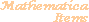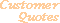Home Older Mathematica Items

Scientific Arts

# Mathematica in Quantum Field Theory Calculations: An Example

## Abstract

The following gives a simple example of the use of Mathematica in the calculation of the one loop "fish" diagram intheory. One approach of many possible ones is given. This is a potential starting point for further investigations by the interested reader.

## Introduction

There are a number of Mathematica tools available for quantum field theory calculations. Several of these are available free of charge from MathSource, and the third party commercial product FeynCalc is available from its developer. However, independent of or in conjunction with these useful tools, Mathematica provides all of the needed functionality for doing quantum field theory calculations from scratch and for implementing the needed transformations and knowledge base of your specific field.

### Problem Set Up

A basic example is the use of dimensional regularization to calculate a one loop diagram intheory. This is the diagram.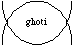The action for thetheory isThe feynman rules for this theory give the following expression for this diagram .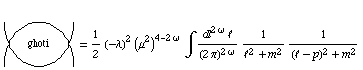The traditional Feynman parameter trick allows us to write the product of the propagators in the form(An example of a function that does this automatically is given in the Appendix.)

When the square is completed in the denominator and the momentum integration variable is changed via the linear transformation, the following integral is obtained (where the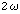-dimensional angular integration has been performed).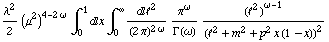### The-Dimensional Integration

``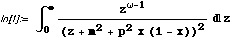``
``

Check to be sure that the requirements of the integration are met.

``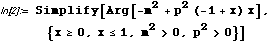``
`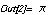`

The dimension of the diagram is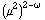, so we pull out a factor of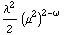so as not to perform any series expansions on this term in the following. We will return it to its rightful place at the end.

Expand the result in a series around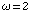to extract the constant and pole parts.

````
`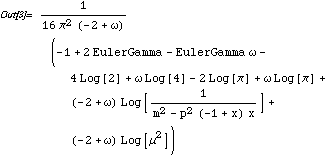`

### Feynman Parameter Integration

We now integrate over the Feynman parameter. We include the option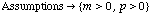to instruct the integration routine about the properties of these parameters.

The raw result that Mathematica returns from this integration is a bit long, so we wrap it in Short and give it the name temporary.

````
``

Perform some simplifications using the same assumptions as in the integration above (note that FullSimplify accepts its assumptions as an argument rather than as an option).

``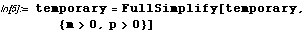``
``

Rearrange terms using pattern matching and replacement rules.

``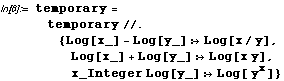``
`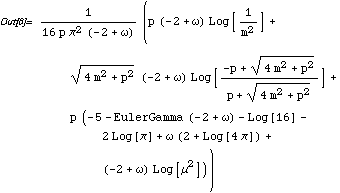`

An easy way to isolate the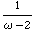pole term is to expand this result in series once again. We use Simplify while we are at it and reintroduce thefactor.

``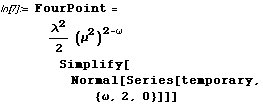``
``

Finally, one small rearrangement to make everything look nice.

````
`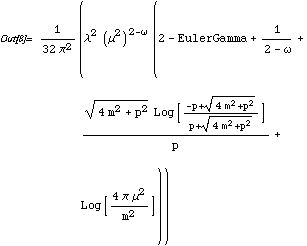`

This is the textbook result. Thus we have, indimensions,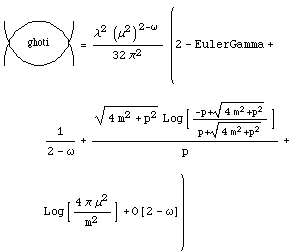## APPENDIX: Feynman Parameterization Function

Here is one way to automatically generate the Feynman parameterization of a product of scalar propagators. The function takes as its first argument a list of denominators and their exponents.  The second argument is a list of integration parameters - one for each denominator.

``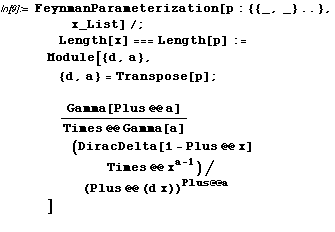``
``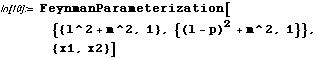``
``
``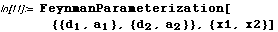``
`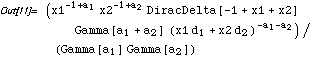`
 For further information on our services send email to info@scientificarts.com . Contents of this web site Copyright © 1999-2011, Scientific Arts, LLC.
 s